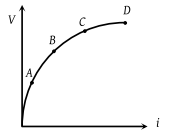Variation of current passing through a conductor as the voltage applied across its ends as varied is shown in the adjoining diagram. If the resistance (R) is determined at the points A, B, C and D, we will find that(1) RC = RD

(2) RB > RA

(3) RC > RB

(4) None of these

(4) From the curve it is clear that slopes at points A, B, C, D have following order A > B > C > D.

And also resistance at any point equals to slope of the V-i curve.

So order of resistance at three points will be ${R}_{A}>{R}_{B}>{R}_{C}>{R}_{D}$

Difficulty Level:

• 13%
• 28%
• 23%
• 38%